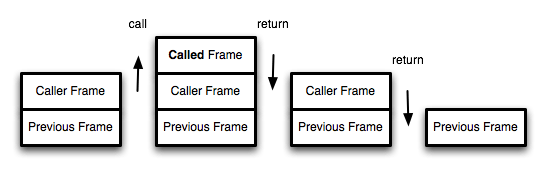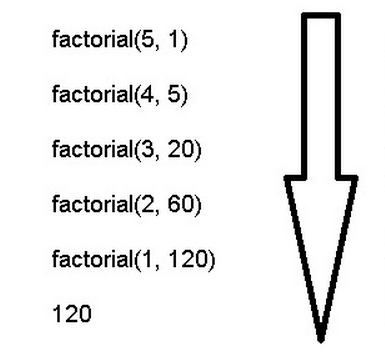# 尾调用优化## 一、什么是尾调用？

``````
function f(x){
return g(x);
}
``````

``````
// 情况一
function f(x){
let y = g(x);
return y;
}

// 情况二
function f(x){
return g(x) + 1;
}
``````

``````
function f(x) {
if (x > 0) {
return m(x)
}
return n(x);
}
``````

## 二、尾调用优化``````
function f() {
let m = 1;
let n = 2;
return g(m + n);
}
f();

// 等同于
function f() {
return g(3);
}
f();

// 等同于
g(3);
``````

## 三、尾递归

``````
function factorial(n) {
if (n === 1) return 1;
return n * factorial(n - 1);
}

factorial(5) // 120
``````

``````
function factorial(n, total) {
return factorial(n - 1, n * total);
}

factorial(5, 1) // 120
``````## 四、递归函数的改写

``````
function tailFactorial(n, total) {
return tailFactorial(n - 1, n * total);
}

function factorial(n) {
return tailFactorial(n, 1);
}

factorial(5) // 120
``````

``````
function currying(fn, n) {
return function (m) {
return fn.call(this, m, n);
};
}

function tailFactorial(n, total) {
return tailFactorial(n - 1, n * total);
}

const factorial = currying(tailFactorial, 1);

factorial(5) // 120
``````

``````
function factorial(n, total = 1) {
return factorial(n - 1, n * total);
}

factorial(5) // 120
``````

（[说明] 本文摘自我写的《ECMAScript 6入门》

## 五、严格模式

ES6的尾调用优化只在严格模式下开启，正常模式是无效的。

• `arguments`：返回调用时函数的参数。
• `func.caller`：返回调用当前函数的那个函数。

（完）

## 留言（45条）

Babel 编译 ES6 生成 ES5尾递归的做法我还是不明白, 用 Object 方法可以绕过调用栈吗?

ES5 递归时写尾调用有效果吗 ?

`引用桑凯的发言：`

ES5 递归时写尾调用有效果吗 ?

function fn(x){
let a=1, b=2;

fn2(1, x);
return;
}

function factorial(n) {
if (n === 1) return 1;
return n * factorial(n - 1);
}

factorial(50000) // 120

`引用oceanstick的发言：`

function factorial(n) {
if (n === 1) return 1;
return n * factorial(n - 1);
}

factorial(50000) // 120

----------------------------------------------------
function factorial(n, total) {
return factorial(n - 1, n * total);
}

factorial(50000, 1)

@冷无缺：

"use strict";
function sum(x, y){
if (y > 0){
return sum(x+1, y-1);
}else{
return x;
}
}

console.log(sum(1, 100000))

@blackcater：看caniuse，目前没有一个浏览器支持，包括Babel。

`引用桑凯的发言：`

`引用笑画戈的发言：`

currying 中返回的是一个方法，这个方法很有可能会被挂载到某个对象上面。所以这里需要用call去改写一下context，防止 currying过程总 context被改写。该例子中确实用不用call都没问题。

function factorial(n) {
'use strict';
var arr = new Array(10000);
if (n === 1) return 1;
return 1 * factorial(n - 1);//这里不是尾调用，需要保存n个调用记录，复杂度 O(n)
}

function _factorial(n, total) {
'use strict';
var arr = new Array(10000);
return _factorial(n - 1, 1 * total);
}

/**
* 没办法从chrome timeline中观察出factorial(10000)、_factorial(10000,1)计算的内存消耗差异，
* 而且factorial(100000)、_factorial(100000,1)都会报错Maximum call stack size exceeded
* 所以我不清楚是否还存在所谓的尾调用优化。
*/

const fun = a => b => a == 0 ? a : fun(b-1)(b+a)

//1.普通递归
function Fibonacci0(n){
if(n return Fibonacci0(n-1)+Fibonacci0(n-2);
}
Fibonacci0(10);//89
Fibonacci0(100);// 卡住了~~~等了很久都没看到执行结果
Fibonacci0(10000);// 同上

//2.改写为尾递归
"use strict";
function Fibonacci1(n,temp=1,result=1){
if(n return Fibonacci1(n-1,result,temp+result);
}
console.log(Fibonacci1(100));//573147844013817200000
console.log(Fibonacci1(10000));//Infinity
console.log(Fibonacci1(1000000));//报错 RangeError: Maximum call stack size exceeded

//3.采用蹦床函数
function Fibonacci2(n,temp=1,result=1){
if(n return Fibonacci2.bind(null,n-1,result,temp+result);
}
//console.log(Fibonacci2(100));
//console.log(Fibonacci2(1000));

//蹦床函数
function trampoline(f){
while (f&&f instanceof Function){
f=f();
}
return f;
}
console.log(trampoline(Fibonacci2(1000000)));//Infinity

@李洋：

----
chrome 断点下在 g 函数中,在 call stack 还是能看到 f 的信息,无论 g 是不是尾调用.

function factorial(n, total) {
return factorial(n - 1, n * total);
}

factorial(5, 1) // 120

```
function factorial(n) {
if (n === 1) return 1;
return n * factorial(n - 1); // 最后一步引用了变量n，so不算尾递归。并且由于引用变量n，so需要保存n个调用记录
}

factorial(5) // 120
```

```
function factorial(n, total) {
return factorial(n - 1, n * total); // 由于未引用变量，so只保留一个调用记录，复杂度 O(1)
}

factorial(5, 1) // 120

```

// 尾递归
"use strict";
function recursion(num){
new_num = num + 1
if (num >= 40000){
return
}
console.log("尾递归|第",new_num,"次调用")
return recursion(new_num)
}

recursion(1)

@昭昭：

V8引擎的尾递归优化已经默认关闭了

`引用昭昭的发言：`

// 尾递归
"use strict";
function recursion(num){
new_num = num + 1
if (num >= 40000){
return
}
console.log("尾递归|第",new_num,"次调用")
return recursion(new_num)
}

recursion(1)

function foo2(data) {
var ret = a(data);
return ret;
}

// 情况一
function f(x){
let y = g(x);
return y;
}

@Jamie：

factorial的时间复杂度是：O(2^n)；
factorial2的时间复杂度是：O(n);

chrome90以上好像不支持“尾调用优化”了，之前还能不报错，现在无论怎么写只要是复杂度够高都会抛错。不过改写之后算法效率会高很多，能支持的复杂度有了提升：比如以前支持1万次嵌套，改写之后能支持到2万次，但是3万依旧会报错。

«-必填

«-必填，不公开

«-我信任你，不会填写广告链接